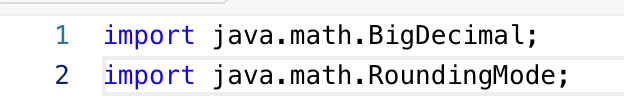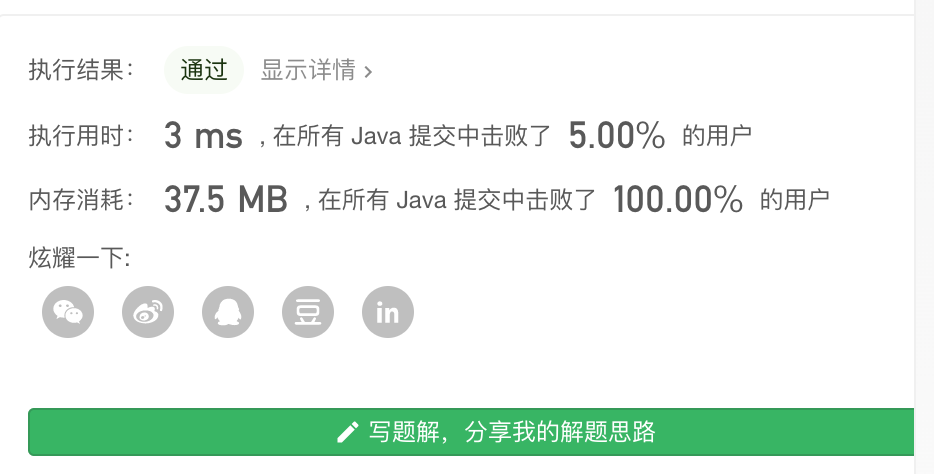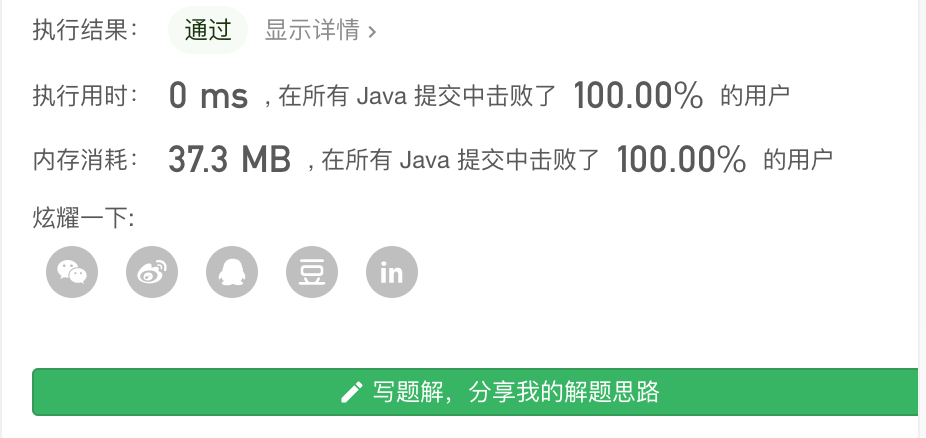示例 1：

输入：salary = [4000,3000,1000,2000]
输出：2500.00000
解释：最低工资和最高工资分别是 1000 和 4000 。


示例 2：

示例 3：

示例 4：

### 提示：

3 <= salary.length <= 100
103 <= salary[i] <= 106
salary[i] 是唯一的。

### 关键点：

1. 计算精度在 10 ^ -5 之内
2. 边界判定

### 实现思路：

1. 定义2个变量，int就够用，用来保存最大值和最小值。
2. 保证计算精度，使用BigDecimal，但是刚开始 leetcode 说找不到变量，于是我手动在 编辑界面增加了导包语句，成功使用，说明 leetcode 是需要手动导包，也是可以导包的。### 代码实现：

  /**
* 1491. 去掉最低工资和最高工资后的平均值
* 关键点：
* 1： 【3 <= salary.length <= 100】 -》 数组长度
* 2：【10^3 <= salary[i] <= 10^6】 元素大小
* 3： 元素唯一
* 4：误差值小于 【10 ^ -5】
* 思路：
* 一个存最大值，一个存最小值
*
* @param salary
* @return
*/
public static double average(int[] salary) {
int max = 0;
int min = 0;
double result = 0.0;
long sum = 0;
for (int i = 0; i < salary.length; i++) {
// 初始化
if (i == 0) {
max = Math.max(salary, salary);
min = Math.min(salary, salary);
i = 1;
continue;
}
if (salary[i] > max) {
max = salary[i];
}

if (salary[i] < min) {
min = salary[i];
}

}
// 循环结束之后 创建一个新的数组，去除最大值和最小值 然后计算平均值
for (int i : salary) {
if (i == max || i == min) {
continue;
}
sum += i;
}
BigDecimal sumD = new BigDecimal(sum);
BigDecimal resultD = sumD.divide(new BigDecimal(salary.length - 2), 5, RoundingMode.HALF_UP);
result = resultD.doubleValue();
System.out.println(result);
return result;
}


### 运行结果：• 说实话这个运行时间 3ms 只击败了 5% 我没看懂为啥
• 37.5 MB 击败了 100% 也没看懂，我猜测可能速度快的解法使用了多个数组？ 我本来也想再创建一个新数组，但是还是使用了遍历的方法。

### 其他人的优秀解法：

1. 对数组进行排序。
2. 遍历数组，去掉第一个和最后一个（最小值和最大值），求和
3. sum / (num.length -2)
 public double average(int[] salary) {
double ans=0;
int len=salary.length;

Arrays.sort(salary);
for(int i=1;i<len-1;i++){
ans+=salary[i];
}
return ans/(len-2);
}自愧不如，Arrays.sort 真是个好api

Q.E.D.